# Orbit Diagrams & PSOS

## Orbit Diagrams of Maps

An orbit diagram (also called bifurcation diagram) is a way to visualize the asymptotic behavior of a map, when a parameter of the system is changed

ChaosTools.orbitdiagramFunction
orbitdiagram(ds::DiscreteDynamicalSystem, i, p_index, pvalues; kwargs...)

Compute the orbit diagram (also called bifurcation diagram) of the given system, saving the i variable(s) for parameter values pvalues. The p_index specifies which parameter of the equations of motion is to be changed.

i can be Int or AbstractVector{Int}. If i is Int, returns a vector of vectors. Else it returns vectors of vectors of vectors. Each entry are the points at each parameter value.

Keyword Arguments

• Ttr::Int = 1000 : Transient steps; each orbit is evolved for Ttr first before saving output.
• n::Int = 100 : Amount of points to save for each initial condition.
• Δt = 1 : Stepping time. Changing this will give you the orbit diagram of the Δt order map.
• u0 = nothing : Specify an initial state. If nothing, the previous state after each parameter is used to seed the new initial condition at the new parameter (with the very first state being the system's state). This makes convergence to the attractor faster, necessitating smaller Ttr. Otherwise u0 can be a standard state, or a vector of states, so that a specific state is used for each parameter.
• ulims = (-Inf, Inf) : only record system states within ulims (only valid if i isa Int).

For example, let's compute the famous orbit diagram of the logistic map:

using DynamicalSystems, CairoMakie

ds = Systems.logistic()
i = 1
pvalues = 3:0.005:4
ics = [rand() for m in 1:10]
n = 2000
Ttr = 2000
p_index = 1
output = orbitdiagram(ds, i, p_index, pvalues; n = n, Ttr = Ttr)

L = length(pvalues)
x = Vector{Float64}(undef, n*L)
y = copy(x)
for j in 1:L
x[(1 + (j-1)*n):j*n] .= pvalues[j]
y[(1 + (j-1)*n):j*n] .= output[j]
end

fig, ax = scatter(x, y; axis = (xlabel = L"r", ylabel = L"x"),
markersize = 0.8, color = ("black", 0.05),
)
ax.title = "total points: $(L*n)" xlims!(ax, pvalues, pvalues[end]); ylims!(ax,0,1) fig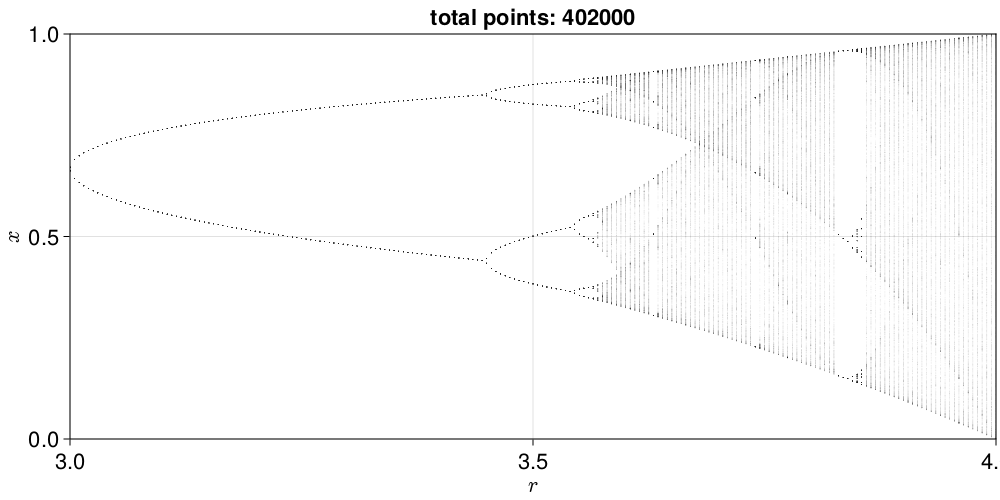Notice that if you are using PyPlot, the plotting process will be slow, since it is slow at plotting big numbers of points. The function is not limited to 1D maps, and can be applied just as well to any discrete system. ## Poincaré Surface of Section Also called Poincaré map is a technique to reduce a continuous system into a discrete map with 1 less dimension. We are doing this using the function: ChaosTools.poincaresosFunction poincaresos(ds::ContinuousDynamicalSystem, plane, tfinal = 1000.0; kwargs...) Calculate the Poincaré surface of section[Tabor1989] of the given system with the given plane. The system is evolved for total time of tfinal. Return a Dataset of the points that are on the surface of section. See also poincaremap for the map version. If the state of the system is$\mathbf{u} = (u_1, \ldots, u_D)$then the equation defining a hyperplane is $$$a_1u_1 + \dots + a_Du_D = \mathbf{a}\cdot\mathbf{u}=b$$$ where$\mathbf{a}, b$are the parameters of the hyperplane. In code, plane can be either: • A Tuple{Int, <: Real}, like (j, r) : the plane is defined as when the jth variable of the system equals the value r. • A vector of length D+1. The first D elements of the vector correspond to$\mathbf{a}$while the last element is$b$. This function uses ds, higher order interpolation from DifferentialEquations.jl, and root finding from Roots.jl, to create a high accuracy estimate of the section. See also produce_orbitdiagram. Notice that poincaresos is just a fancy wrapper of initializing a poincaremap and then calling trajectory on it. Keyword Arguments • direction = -1 : Only crossings with sign(direction) are considered to belong to the surface of section. Positive direction means going from less than$b$to greater than$b$. • idxs = 1:dimension(ds) : Optionally you can choose which variables to save. Defaults to the entire state. • Ttr = 0.0 : Transient time to evolve the system before starting to compute the PSOS. • u0 = get_state(ds) : Specify an initial state. • warning = true : Throw a warning if the Poincaré section was empty. • rootkw = (xrtol = 1e-6, atol = 1e-6) : A NamedTuple of keyword arguments passed to find_zero from Roots.jl. • diffeq is a NamedTuple (or Dict) of keyword arguments propagated into init of DifferentialEquations.jl. See trajectory for examples. Only valid for continuous systems. poincaresos(A::Dataset, plane; kwargs...) Calculate the Poincaré surface of section of the given dataset with the given plane by performing linear interpolation betweeen points that sandwich the hyperplane. Argument plane and keywords direction, warning, idxs are the same as above. Here is an example of the Henon-Heiles system showing the mixed nature of the phase space using DynamicalSystems, CairoMakie hh = Systems.henonheiles() plane = (1, 0.0) u0s = [[0.0, -0.25, 0.42081, 0.0], [0.0, -0.31596, 0.354461, 0.0591255], [0.0, 0.1, 0.5, 0.0], [0.0, -0.0910355, 0.459522, -0.173339], [0.0, -0.205144, 0.449328, -0.0162098]] fig = Figure(resolution = (500,500)) ax = Axis(fig[1,1]; xlabel = L"q_2", ylabel = L"p_2") for u0 in u0s psos = poincaresos(hh, plane, 20000.0; u0 = u0) scatter!(ax, psos[:, 2], psos[:, 4]; markersize = 2.0) end fig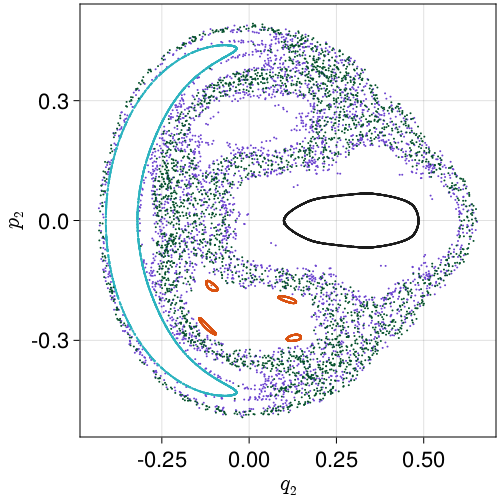Here the surface of section was the (hyper-) plane that$q_1 = 0$. Some chaotic and regular orbits can be seen in the plot. You can tell the regular orbits apart because they look like a single connected curve. This is the result of cutting a 2-torus by a plane! ### Advanced hyperplane Finally here is one more example with a more complex hyperplane: gis = Systems.gissinger([2.32865, 2.02514, 1.98312]) # Define appropriate hyperplane for gissinger system const ν = 0.1 const Γ = 0.9 # default parameters of the system # I want hyperperplane defined by these two points: Np(μ) = SVector{3}(sqrt(ν + Γ*sqrt(ν/μ)), -sqrt(μ + Γ*sqrt(μ/ν)), -sqrt(μ*ν)) Nm(μ) = SVector{3}(-sqrt(ν + Γ*sqrt(ν/μ)), sqrt(μ + Γ*sqrt(μ/ν)), -sqrt(μ*ν)) # Create hyperplane passing through Np, Nm and 0: using LinearAlgebra gis_plane(μ) = [cross(Np(μ), Nm(μ))..., 0] μ = 0.119 set_parameter!(gis, 1, μ) fig = Figure() ax = Axis3(fig[1,1]; xlabel="Q", ylabel="D", zlabel="V") psos = poincaresos(gis, gis_plane(μ), 10000.0, Ttr = 200.0) scatter!(ax, columns(psos)...) fig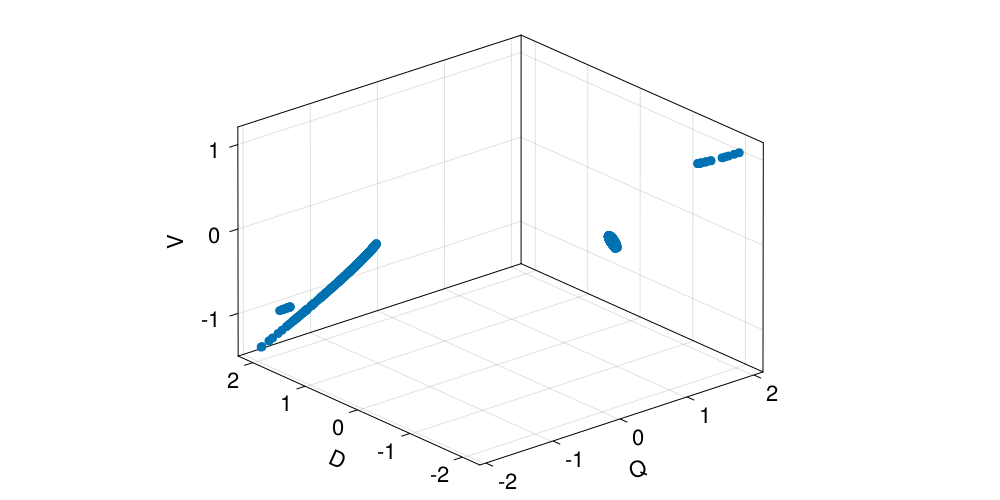### Stroboscopic Map A special case of a PSOS is a stroboscopic map, which is defined for non-autonomous systems with periodic time dependence, like e.g. the Systems.duffing oscillator. A "cut" through the phase-space can be produced at every period$T = 2\pi/\omega\$. There is no reason to use poincaresos for this though, because you can simply use trajectory and get the solution with a certain time sampling rate. For example, this piece of code:

using DynamicalSystems, Plots

ds = Systems.duffing(β = -1, ω = 1, f = 0.3) # non-autonomous chaotic system

frames=120
a = trajectory(ds, 100000.0, Δt = 2π/frames, Ttr=20π) # every period T = 2π/ω

orbit_length = div(size(a), frames)
a = Matrix(a)

@gif for i in 1:frames
orbit_points = i:frames:(orbit_length*frames)
scatter(a[orbit_points, 1], a[orbit_points, 2], markersize=1, html_output_format=:png,
leg=false, framestyle=:none, xlims=extrema(a[:,1]), ylims=extrema(a[:,2]))
end

Produces this nice animation: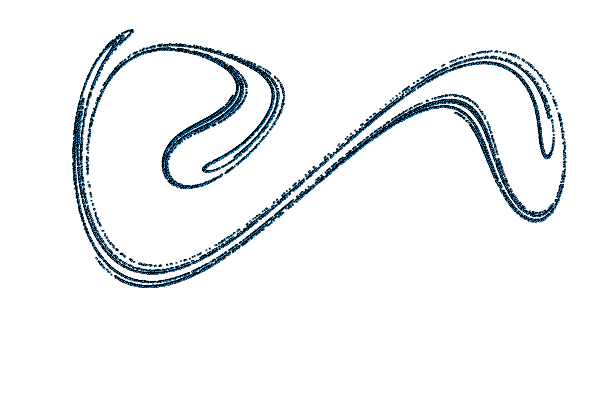## Producing Orbit Diagrams for Flows

The orbitdiagram does not make much sense for continuous systems, besides the trivial case where the system is at a fixed point. In order for orbitdiagram to have meaning one must have a map.

If only there was a way to turn a continuous system into a map... OH WAIT! That is what poincaresos does! By performing successive surfaces of section at different parameter values, one can indeed "produce" an orbit diagram for a flow.

We have bundled this process in the following function:

ChaosTools.produce_orbitdiagramFunction
produce_orbitdiagram(
ds::ContinuousDynamicalSystem, plane, i::Int, p_index, pvalues; kwargs...
)

Produce an orbit diagram (sometimes wrongly called bifurcation diagram) for the i variable(s) of the given continuous system by computing Poincaré surfaces of section using plane for the given parameter values (see poincaresos).

i can be Int or AbstractVector{Int}. If i is Int, returns a vector of vectors. Else it returns a vector of vectors of vectors. Each entry are the points at each parameter value.

Keyword Arguments

• printparams::Bool = false : Whether to print the parameter used during computation in order to keep track of running time.
• direction, warning, Ttr, rootkw, diffeq : Propagated into poincaresos.
• u0 = nothing : Specify an initial state. If nothing, the previous state after each parameter is used to seed the new initial condition at the new parameter (with the very first state being the system's state). This makes convergence to the attractor faster, necessitating smaller Ttr. Otherwise u0 can be a standard state, or a vector of states, so that a specific state is used for each parameter.

Description

For each parameter, a PSOS reduces the system from a flow to a map. This then allows the formal computation of an "orbit diagram" for the i variable of the system, just like it is done in orbitdiagram.

The parameter change is done as p[p_index] = value taking values from pvalues and thus you must use a parameter container that supports this (either Array, LMArray, dictionary or other).

For example, we will calculate the orbit diagram of the Shinriki oscillator, a continuous system that undergoes a period doubling route to chaos, much like the logistic map!

ds = Systems.shinriki([-2, 0, 0.2])

pvalues = range(19, stop = 22, length = 201)
i = 1
plane = (2, 0.0)
tf = 200.0
p_index = 1

output = produce_orbitdiagram(ds, plane, i, p_index, pvalues;
tfinal = tf, Ttr = 200.0)

fig = Figure()
ax = Axis(fig[1,1]; xlabel = L"R_1", ylabel = L"V_1")
for (j, p) in enumerate(pvalues)
scatter!(ax, fill(p, length(output[j])), output[j];
color = ("black", 0.5), markersize = 1
)
end
fig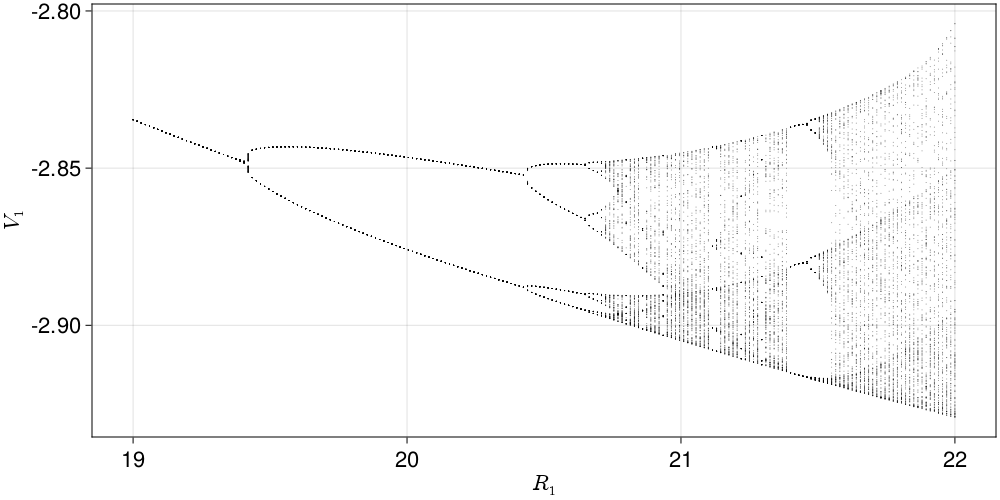• Tabor1989M. Tabor, Chaos and Integrability in Nonlinear Dynamics: An Introduction, §4.1, in pp. 118-126, New York: Wiley (1989)